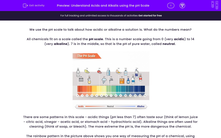# Understand Acids and Alkalis using the pH Scale

In this worksheet, students will learn about the quantitative description of acids and alkalis using the pH scale.Key stage:  KS 4

Year:  GCSE

GCSE Boards:   AQA, AQA Trilogy, AQA Synergy, OCR 21st Century, OCR Gateway, Pearson Edexcel, Eduqas,

Difficulty level:#### Worksheet Overview

We use the pH scale to talk about how acidic or alkaline a solution is. What do the numbers mean?

All chemicals fit on a scale called the pH scale. This is a number scale going from 0 (very acidic) to 14 (very alkaline). 7 is in the middle, so that is the pH of pure water, called neutralThere are some patterns in this scale - acidic things (pH less than 7) often taste sour (think of lemon juice - citric acid, vinegar - acetic acid, or stomach acid - hydrochloric acid). Alkaline things are often used for cleaning (think of soap, or bleach). The more extreme the pH is, the more dangerous the chemical.

The rainbow pattern in the picture above shows you one way of measuring the pH of a chemical, using universal indicator. We can have universal indicator as a liquid, or we can make paper with universal indicator soaked into it.

If we add this to some chemical, the colour of the indicator will change to indicate the pH of the chemical. Universal indicator isn't very precise, but it gives us a rough idea of the pH of a solution. We can get much more accurate results using an electronic pH meter.The thing that determines if a solution is acidic or alkaline is the presence of H+ ions or OH- ions. Acidic solutions have excess H+ ions dissolved in them. Alkaline solutions have excess OH- ions dissolved in them.

The pH value is linked to the concentration of hydrogen ions in a solution - one step on the pH scale (so from 5 to 4, or 3 to 2) means the concentration of H+ ions increases by a factor of ten. This type of scale is called logarithmic.

Both H+ ions and OH- ions are very reactive, which is why both acids and alkalis are potentially harmful.

However, if H+ and OH- combine, they make H2O, which is water. That's why we can use acid to neutralise alkali, and alkali to neutralise acid.

Ionic equations

When we dissolve acid in water, it releases H+ ions.

For example, we make hydrochloric acid by dissolving hydrogen chloride in water:

HCl (s) → H+ (aq) + Cl-( aq).

We make alkalis by dissolving sources of hydroxide in water.

For example, we can dissolve sodium hydroxide in water: NaOH (s) → Na+ (aq) + OH- (aq).

Neutralisation involves the reaction H+ (aq) + OH- (aq) → H2O (l).

Strong and weak acids, dilute and concentrated acids

The concentration of H+ ions depends on two factors.

One is the concentration of the solute in water. A solution with a large mass of solid acid and a small volume of water will increase the concentration of H+ ions, leading to a lower (more extreme) pH.

A solution with a small mass of solid acid and a large volume of water is called a dilute solution. The more dilute the solution, the lower the concentration of H+ ions, leading to a pH nearer 7.

The other factor is the strength of the acid. A strong acid fully releases H+ ions into solution when dissolved. A weak acid only partially releases H+ ions into solution. Strong acids have lower (more extreme) pH than weak acids.

Let's move on to some questions now.

### What is EdPlace?

We're your National Curriculum aligned online education content provider helping each child succeed in English, maths and science from year 1 to GCSE. With an EdPlace account you’ll be able to track and measure progress, helping each child achieve their best. We build confidence and attainment by personalising each child’s learning at a level that suits them.

Get started